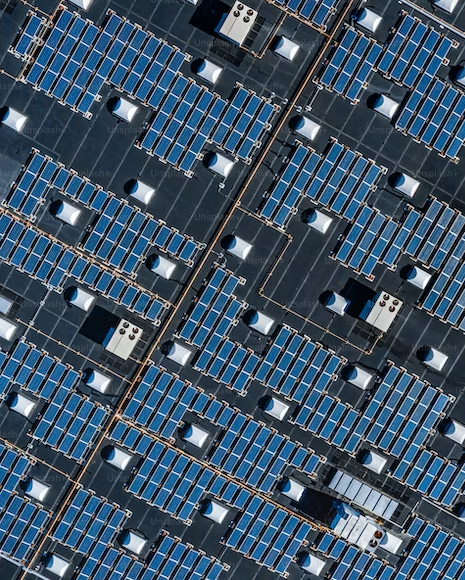Email : phmtools@gmail.com         WhatsApp: +8613780298990
English

# Decoding the Power of 0.5C in Energy Storage SystemsUsually, when discussing the scale of an energy storage system, we use the term "power/energy" to represent it. In energy storage projects, we often encounter expressions like 1C (1-hour system), 0.5C (2-hour system), 0.25C (4-hour system) to indicate the system's capacity. In the context of energy storage systems, "C" refers to the discharge rate of the battery, which measures the speed of discharging. The charge/discharge rate is calculated as the ratio of charge/discharge current to the rated capacity. For example, if a battery with a capacity of 100A·h discharges at 15A, its discharge rate is 0.15C.Let's understand the algorithm with an example: a 100Ah battery discharging at 1C means discharging at a current of 100A, and 2C means discharging at a current of 200A. Similarly, 0.5C (or 1/2C) means discharging at 50A, and 0.2C (or 1/5C) means discharging at 20A. The charge rate follows the same principle. So, "C" represents the magnitude of charge and discharge current for general batteries. In solar PV and storage systems, we often see expressions like "10%*2h" where the "10%" refers to the storage ratio, meaning the storage capacity is 10% of the newly added PV capacity, and the "2h" indicates the continuous discharge duration, representing the storage device's ability to discharge continuously for at least 2 hours at the rated power. The charge/discharge rate "C" is used to measure the charge and discharge duration. For example, a 100MW solar PV system with a 10%*2h storage configuration will have an energy storage station of 10MW/20MWh, where 10MW represents the rated power and 20MWh represents the energy capacity. In this case, if the discharge is done at the rated power of 10MW, the station will be able to discharge all the energy in 2 hours, indicating a charge/discharge rate of 0.5C. By knowing the charge/discharge rate of the energy storage system, we can determine the time required for charging and discharging. For instance, a charge/discharge rate of 1C means the energy storage battery can discharge all its capacity within 1 hour, while 0.5C means it can discharge all its capacity within 2 hours.

Article May Be Of Interest To You
Why Choose Lithium Valley
7*24 Hours Service
Lithium-ion batteries power all sorts of devices – power tools, notebook computers, tablets, cell phones, and electric cars. They have some distinct advantages over wet-cell lead-acid batteries, such as you’d find in your car. The rapid drop in prices and evolution of lithium-ion batteries and associated technology is changing all that. The new generation of solar energy storage is cheaper to buy, more cost-effective, streamlined, and better looking.​​​​​​​
  Lighter
  Higher energy density
  Lower self-discharge
  Lower maintenance
  No “memory effect”
  Increased cycle life
Sales@lithiumvalley.com
+86 13423188746
If You Still Have Questions

Ask Us For Service And Technical Support